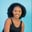Related Tags

python
numpy

# How to find the sum and product of a 2-D array in PythonMaria Elijah

## Overview

The Python library NumPy has the methods sum() and product(), which can be used to find the sum and product of array elements.

### The numpy.sum() method

#### Syntax

numpy.sum(arr, axis=None, dtype=None, out=None)


#### Return value

The numpy.sum() method returns the sum of elements in a given input array over a specified axis.

### The numpy.prod() method

#### Syntax

numpy.prod(arr, axis=None, dtype=None, out=None)


#### Return value

The numpy.prod() method returns the product of elements in a given input array over a specified axis.

Note: A 2-D array can be created using a list of lists in Python.

### Parameters

• arr: This is an array_like object that represents the input data.
• axis: This is an optional parameter. It represents the axes along which the operation is to be performed.
• dtype: This is an optional parameter. It represents the return type of the array.
• out: This is an optional parameter. It represents the alternative output array in which the result is to be placed.

Note: If the out parameter is specified, it returns an array reference to out.

### Examples

The following code snippets show how to find the sum and product of the 2-D array using the numpy.sum() and numpy.prod() methods.

#### Example 1

Find the sum of a 2-D array using the numpy.sum() method.

# import numpy
import numpy as np
# create a list
my_list1 = [24,8,3,4,34,8]
my_list2 = [5,7,2,10,15,7]
# convert to a numpy 2D array
np_list = np.array([my_list1, my_list2])
# compute the sum of array elements and store it
np_list_sum = np.sum(np_list, axis=0)

print(np_list_sum)
Calculating the sum of a 2D array

#### Explanation

• Line 2: We import the numpy library.
• Line 4–5: We create two lists called my_list1 and my_list2.
• Line 7: We convert the lists to a NumPy array which gives us a 2-D array. Then, the value is stored in a variable called np_list.
• Line 9: We use the np.sum() to compute the sum of elements in the np_list.
• Line 11: We display the result.

#### Example 2

Find the product of a 2-D array using the numpy.product() method.

# import numpy
import numpy as np
# create a list
my_list1 = [24,8,3,4,8]
my_list2 = [5,7,2,10,7]
# convert to a numpy 2D array
np_list = np.array([my_list1, my_list2])
# compute the product of 2D array
# and store the result in np_list_cumprod
np_list_prod = np.prod(np_list, axis=0)

print(np_list_prod)
Calculating the product of a 2-D array

#### Explanation

• Line 2: We import the numpy library.
• Line 4–5: We create two lists called my_list1 and my_list2.
• Line 7: We convert the lists to a NumPy array which gives us a 2-D array. Then, we store the value in a variable called np_list.
• Line 10: We use the np.prod() to compute the product of elements in the np_list.
• Line 11: We display the result.

RELATED TAGS

python
numpy

CONTRIBUTORMaria Elijah
RELATED COURSES

View all Courses

Keep Exploring

Learn in-demand tech skills in half the time